# Pandas DataFrame Plot boxplot graph

Pandas plot

Pandas.DataFrame.plot to generate boxplot using data

Box plot display summary of five that is minimum, first quartile, median, third quartile and maximum.
The box is drawn between first and third quartile.
Median is drawn within the box.
Let us try one example. Here we have sample data for two subjects.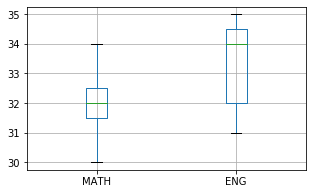``````import pandas as pd
my_dict={
'MATH':[30,33,32,32,31,34,32],
'ENG':[32,31,35,34,35,34,32]
}
df = pd.DataFrame(data=my_dict)
df.boxplot(figsize=(5,3),grid=True)``````

## boxplot with options

There are several options we can add to above boxplot.

## return_type

Default type is 'axis', we can set it to return_type='dict' , return_type='axes' or return_type='both'.

## grid

We will show grid ( grid=True ) or not ( grid=False)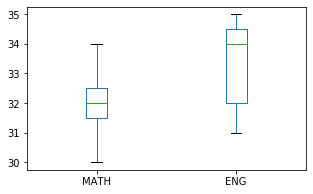``df.boxplot(grid=False,figsize=(5,3))``

## column

If your source file has more columns and you want to specify the column to use.
``df.boxplot(column='Open Price',figsize=(10,4),grid=True)``
More than one coloumn
``df.boxplot(column=['Close Price','Open Price'],grid=True)``

## figsize :

Size of the graph , it is a tuple saying width and height in inches, figsize=(6,3). Here width is 6 inches and height is 3 inches.
``df.boxplot(figsize=(5,3))``

## rot

rot=45 Rotation of x axis labels in degree.
Rotation of ticks ( check the names at x axis )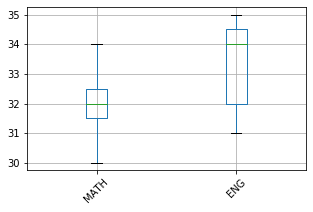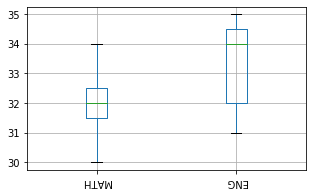``df.boxplot(figsize=(5,3),rot=180)``

## fontsize

fontsize=20 , we can set the font size used labels in x and y axis.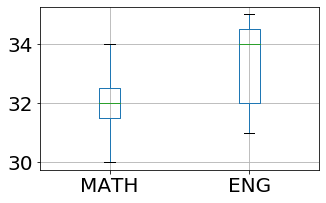``df.boxplot(figsize=(5,3),fontsize=20)``

Subscribe to our YouTube Channel here

## Subscribe

* indicates required
Subscribe to plus2netplus2net.com